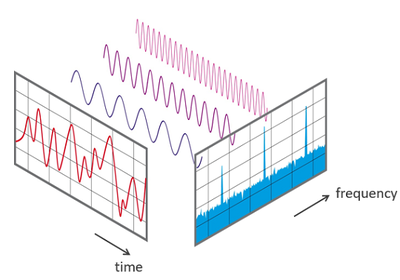# Fourier-Transforms## An Intuitive Interpretation Of The Fourier Transform (or The Link Between Fourier Analysis And Linear Algebra)

My objective in this post is to show a way of understanding the Fourier transform from a linear algebraic perspective. Once an appreciation for this connection is gained, many of the subtleties of the Fourier transform become almost obvious when observed through this lens

Posted

#Linear-Algebra #Fourier-Transforms